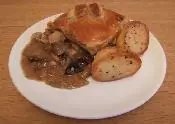# `.queue()`S2C Home « Effects « `.queue()`

Function queues.

## Description

The `.queue()` method is used to show or manipulate the function queue to be executed on the matched set.

• The default queue is named 'fx' and has certain properties that are not shared with named queues:
1. The 'fx' queue will automatically `.dequeue()` the next function on the queue and run it if the queue hasn't started.
2. When `.dequeue()` is used on a function from the 'fx' queue, it will `unshift()` the string 'inprogress', into the  location of the array, thus flagging that the 'fx' queue is currently executing.
3. Being the default, the 'fx' queue is called by `.animate()` and any other function that calls it by default.

## Syntax

Signature Description
`.queue( [queueName] )`Show the function queue, optionally using a queue name to identify the queue.
`.queue( [queueName], newQueue )`Manipulate the queue of functions to be executed on the matched elements, optionally using a queue name to identify the queue.
`.queue( [queueName], callback( next ) )`Use a callback to manipulate the queue of functions to be executed on the matched elements, optionally using a queue name to identify the queue.

## Parameters

Parameter Description Type
`queueName`A string containing the name of the queue. Defaults to 'fx', which is the standard effects queue. `String`
`newQueue`An array of functions to replace the current queue contents.`Array`
`callback( next )`The new function to add to the queue, with a function to call that will dequeue the next item. `Function`

## Return

A JavaScript `Array` object.

#### `.queue( [queueName] )` ExamplesTop

Show the function queue to be executed on the matched set.

In the example below when the button is pressed we move the image below of korma around the screen using the default 'fx' queue. We display the queue length and also the the action on the currently animating action which will have the value 'inprogress'.

``````
\$(function(){
\$('#btn3').on('click', function() {
animateImg1(\$('#korma'));
showQueueFx();
});
function animateImg1(anImg) {
anImg.animate({left:'+=610'},1500);
anImg.animate({top:'+=50'},1500);
anImg.slideToggle(1000);
anImg.slideToggle("fast");
anImg.animate({left:'-=610'},1000);
anImg.animate({top:'-=50'},1500);
}
function showQueueFx() {
var fxQueue = \$('#korma').queue('fx');
\$('#animspan1').text( fxQueue.length );
\$('#animspan2').text( fxQueue );
setTimeout(showQueueFx, 100);
}
});

``````Default queue (fx). The queue length is: . Currently animating function:

Press the buttons below to action the above code:

In the example below when the button is pressed we dequeue one item from the queue named 'curry'. Keep clicking the button to see each animation.

``````
\$(function(){
\$('#masala').queue('curry', function() {
\$(this).animate({right:"+=410"},1500);
});
\$('#masala').queue('curry', function() {
\$(this).slideToggle(1000);
});
\$('#masala').queue('curry', function() {
\$(this).slideToggle("fast");
});
\$('#masala').queue('curry', function() {
\$(this).animate({right:"-=410"},1000);
});
\$('#btn4').on('click', function() {
var \$curryQueue = \$('#masala').queue('curry');
\$('#masala').dequeue('curry');
\$('#animspan3').text( \$curryQueue.length );
});
});

``````Named queue (curry).The queue length is: .

Press the buttons below to action the above code:

#### `.queue( [queueName], newQueue )` ExampleTop

Manipulate the queue of functions to be executed on the matched elements.

In the example below when the button is pressed we add two animations to the queue named 'curry2' and then dequeue them.

``````
\$(function(){
\$('#btn5').on('click', function() {
\$('#thai').queue('curry2', [function(){\$('#thai').slideToggle(1000);},
function(){\$('#thai').slideToggle("fast");}]);
\$('#thai').dequeue('curry2');
\$('#thai').dequeue('curry2');
});
});

``````Press the buttons below to action the above code:

#### `.queue( [queueName], callback( next ) )` ExamplessTop

Use a callback to manipulate the queue of functions to be executed on the matched elements.

In the example below when the button is pressed we push elements from an array onto a queue we have created 'ourQueue'. We then pass this queue through a callback function, dequeueing each item to animate the image.

``````
\$(function(){
\$('#btn6').on('click', function() {
var ourQueue = \$({}), // jQuery on an empty object - a perfect queue holder
queueArray = [function(){\$('#chicken').slideToggle(1000);},
function(){\$('#chicken').slideToggle("fast");}];
\$.each(queueArray, function(index, element) {
ourQueue.push(element);
});
ourQueue.queue(function(next) {
\$(this).dequeue();
next();
});
});
});

``````Press the buttons below to action the above code:

## Related Tutorials

jQuery Intermediate Tutorials - Lesson 8 - Controlling Effects